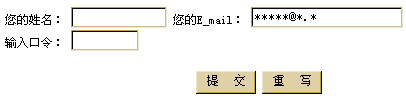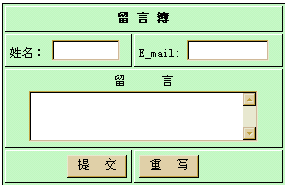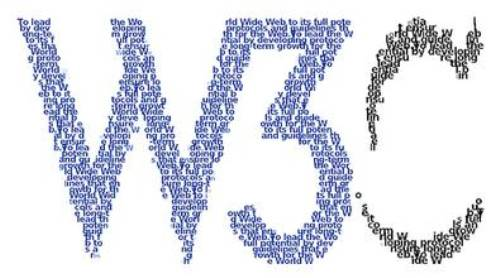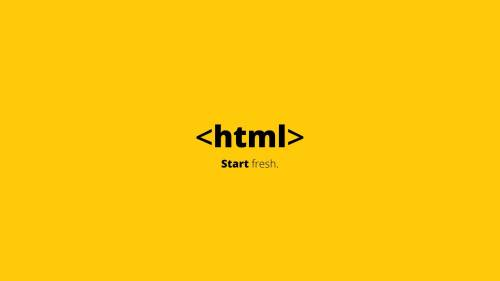# HTML文本框参考样式及常见操作技巧大全

--------------------------------------------------------------

W3Cschool文本输入框实例：

--------------------------------------------------------------

``````<p>
W3Cschool文本输入框实例：<input type="text" value="请输入文本" >
</p>``````

``````.mytxt {
color:#333;
line-height:normal;
font-family:"Microsoft YaHei",Tahoma,Verdana,SimSun;
font-style:normal;
font-variant:normal;
font-stretch:normal;
font-weight:normal;
margin-top:0px;
margin-bottom:0px;
margin-left:0px;
font-size:15px;
outline-width:medium;
outline-style:none;
outline-color:invert;
background-attachment:scroll;
background-repeat:repeat-x;
background-position-x:left;
background-position-y:top;
background-size:auto;
background-clip:border-box;
background-color:rgb(255,255,255);
margin-right:8px;
border-top-color:#ccc;
border-right-color:#ccc;
border-bottom-color:#ccc;
border-left-color:#ccc;
border-top-width:1px;
border-right-width:1px;
border-bottom-width:1px;
border-left-width:1px;
border-top-style:solid;
border-right-style:solid;
border-bottom-style:solid;
border-left-style:solid;
}```
```

<input type="text" value="" class="mytxt" />

``````.mytxt:focus {
border: 1px solid #fafafa;

}
``````

## 一、一个单行文本框的例子``````<form name="form1" action="mailto:3400982550@qq.com" method="post" enctype="text/plain">
<p>您的姓名： <input type="text" name="text1" size="12" maxlength="20">

<p align="center">
<input type="submit" name="提交" value="提 交">
<input type="reset" name="重写" value="重 写">
</p>
</form>
``````## 二、检验用户输入的信息

``````<script language="javascript">
<!--
function test(form){
test1(form);
test2(form);
test3(form)}
function test1(form){
if (form.text1.value=="")
}
function test2(form){
if (form.text2.value==""||form.text2.value.indexOf('@',0)==-1)
}
function test3(form){
if (form.text3.value！="12345678")
}
-->
</script>
``````

## 三、制作一个留言簿``````<form name="form2" action="mailto:fyy0528@sina.com" method="post" enctype="text/plain" >
<table width="50%" border="1" bordercolorlight="#000000" bordercolordark="#FFFFFF" bgcolor="#CCFFCC" cellpadding="4" align="left">
<tr>
<td colspan="2"> <div align="center"><b>留 言 簿</b></div> </td>
</tr>
<tr>
<td>姓名： <input type="text" name="textfield" size="8"> </td>
<td>E_mail: <input type="text" name="textfield2" size="10" maxlength="20"> </td>
</tr>
<tr>
<td colspan="2"> <div align="center">留 言<br> <textarea name="textfield3" cols="30" rows="3"></textarea>
</div>
</td>
</tr>
<tr>
<td> <div align="right"> <input type="submit" name="Submit" value="提 交"> </div> </td>
<td> <input type="reset" name="Submit2" value="重 写"> </td>
</tr>
</table>
</form>
``````

## html文本框参考样式

``````<input style="background:transparent;border:1px solid #ffffff">
``````

``````<INPUT value="Type here" NAME="user_pass" TYPE="text" SIZE="29" onmouseover="this.style.borderColor='black';this.style.backgroundColor='plum'"
style="width: 106; height: 21"
onmouseout="this.style.borderColor='black';this.style.backgroundColor='#ffffff'" style="border-width:1px;border-color=black">``````

``````<Script Language="JavaScript">
function borderColor(){
if(self['oText'].style.borderColor=='red'){
self['oText'].style.borderColor = 'yellow';
}else{
self['oText'].style.borderColor = 'red';
}
oTime = setTimeout('borderColor()',400);
}
</Script>
<input type="text" id="oText" style="border:5px dotted red;color:red" onfocus="borderColor(this);" onblur="clearTimeout(oTime);">
``````

``````<style>
#oText{border:1px dotted #ff0000;ryo:expression_r(onfocus=function light (){with(document.all.oText){style.borderColor=(style.borderColor=="#ffee00"?"#ff0000":"#ffee00");timer=setTimeout(light,500);}},onblur=function(){this.style.borderColor="#ff0000";clearTimeout(timer)})};
</style>
<input type="text" id="oText">
``````

``````<input type="text" style="huerreson:expression_r(this.width=this.scrollWidth)" value="abcdefghijk">
``````

``````<textarea name="content" rows="6" cols="80" onpropertychange="if(this.scrollHeight>80) this.style.posHeight=this.scrollHeight+5">输入几个回车试试</textarea>
``````

``````<input style="border:0;border-bottom:1 solid black;background:;">
``````

``````<script for="T" event="onkeyup">
if(value.length==3)document.all[event.srcElement.sourceIndex+1].select();
</script>
<input name="T" size="5" maxlength="3">—<input name="T" size="5" maxlength="3">—<input name="T" size="5" maxlength="3">—<input name="T" size="5" maxlength="3">—<input name="T" size="5" maxlength="3">—<input name="T7" size="5" maxlength="3">
``````

``````<script for="T" event="onkeyup">if(value.length==maxLength)document.all[event.srcElement.sourceIndex+1].focus();</script>
<script for="T" event="onfocus">select();</script>
<script for="Submit" event="onclick">
var sn=new Array();
for(i=0;i<T.length;i++)
sn=T.value;
</script>
<input name="T" size="5" maxlength="3">—<input name="T" size="5" maxlength="3">—<input name="T" size="5" maxlength="3">—<input name="T" size="5" maxlength="3">—<input name="T" size="5" maxlength="3">—<input name="T" size="5" maxlength="3">
<input type="submit" name="Submit">
``````

## html文本框常见操作技巧

1、html文本框怎么用css变圆角

border-radius: 1-4 length|% / 1-4 length|%;2、HTML中如何设置文本框的大小

<textarea id="txtCon" >content</textarea>
<style type="text/css">
#txtCon{width:100px; height:20px;}
</style>

<textarea id="txtCon" rows="100" cols="100" >content</textarea>

3、在html中如何实现将本网页中文本框中的值传递到另一个网页的用户名密码框中，并实现登陆

<SCRIPT language=JavaScript>
var url = window.location.href;

< /SCRIPT>4、HTML中让表单input等文本框为只读不可编辑的方法

<input type="text" name="input1" value="中国" onfocus=this.blur()>

<input type="text" name="input1" value="中国" disabled="true">

<input name="ly_qq" type="text" tabindex="2" onMouseOver="this.className='input_1'" onMouseOut="this.className='input_2'" value="123456789" disabled="true"　readOnly="true" />

disabled="true" 此果文字会变成灰色，不可编辑。

css屏蔽输入：<input style="ime-mode: disabled">

5、您还可以为您将要创建的文本框预设置一个想要显示的值，具体的设置方法您可以参考本站的编程测试

## 您可能还喜欢：

App下载